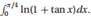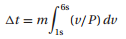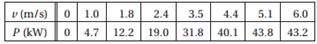Create an Account

Home / Questions / 1 Use the recursive trapezoidal rule to evaluate Explain the results 2 The table shows t...

1 Use the recursive trapezoidal rule to evaluate Explain the results 2 The table shows the power P supplied to the driving wheels of a car as a function of the speed v If the mass of the car is

1. Use the recursive trapezoidal rule to evaluateExplain the results.

2. The table shows the power P supplied to the driving wheels of a car as a function of the speed v. If the mass of the car is m= 2000 kg, determine the time t it takes for the car to accelerate from1m/s to 6 m/s. Use the trapezoidal rule for integration. Hint:which can be derived from New ton’s law F = m(dv/dt) and the definition of power P = Fv.Jul 27 2020 View more View LessSubscribe To Get Solution